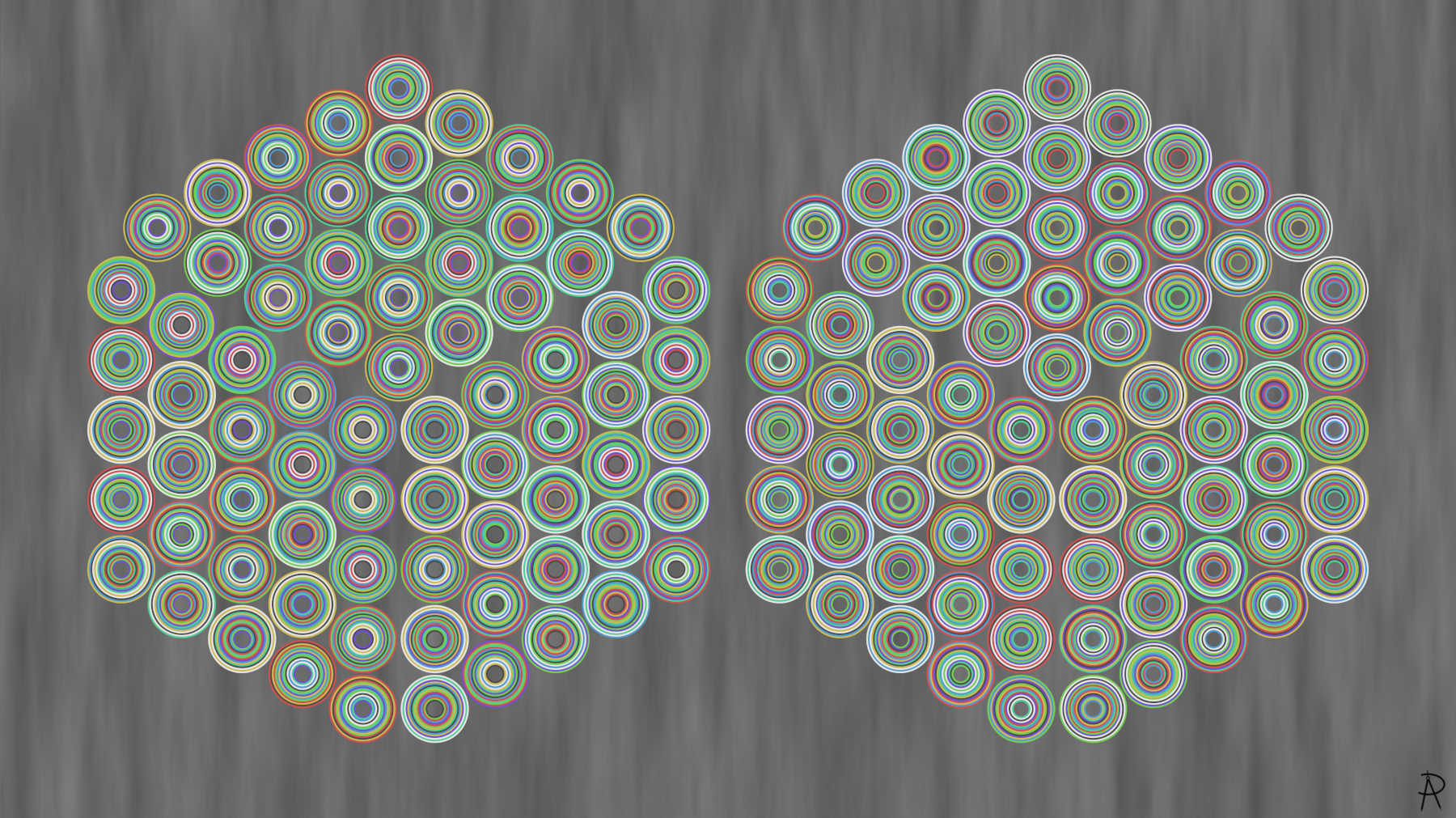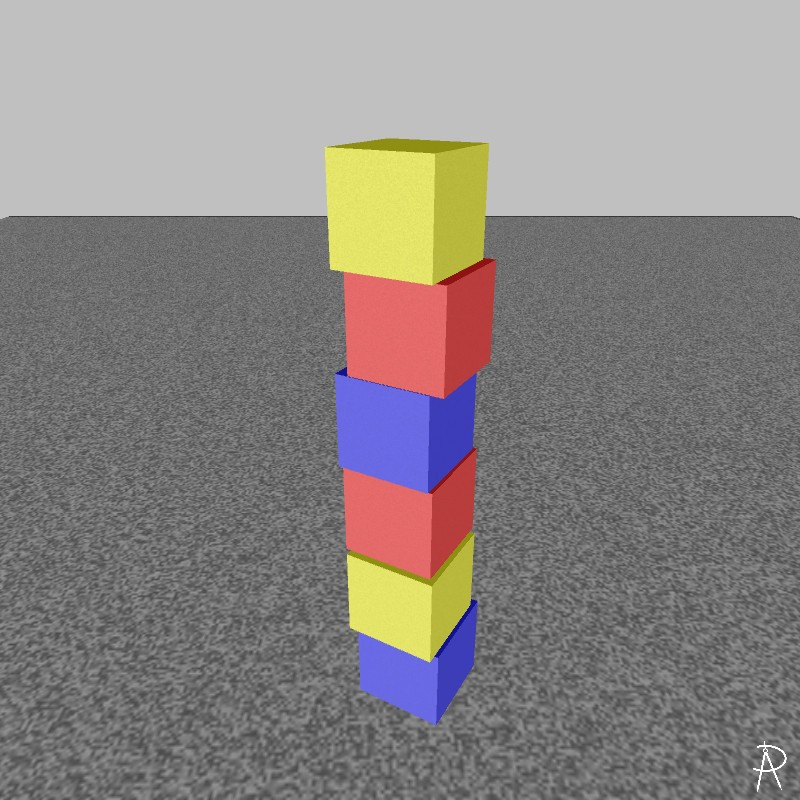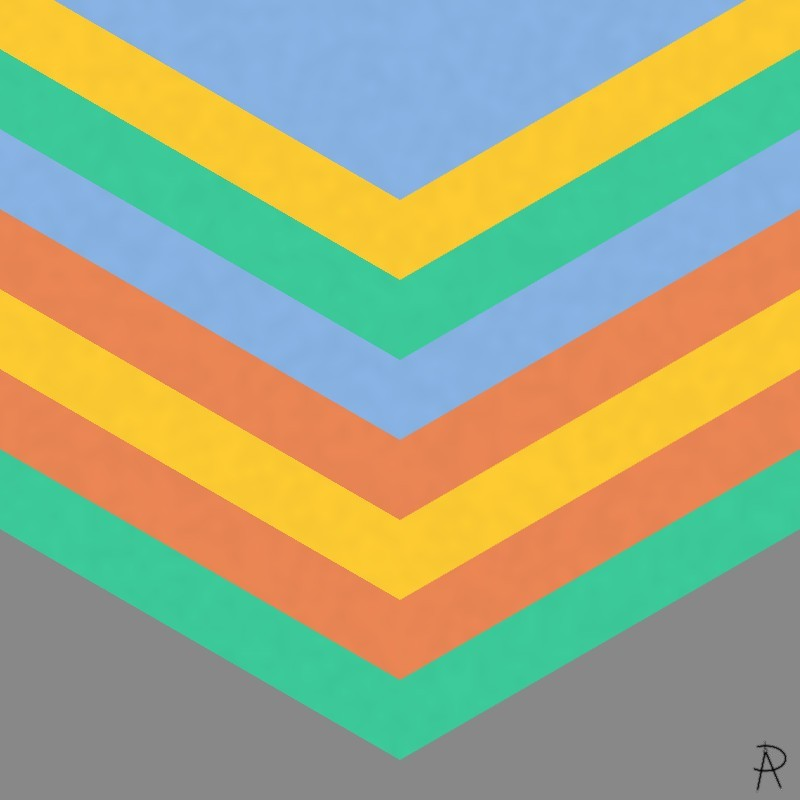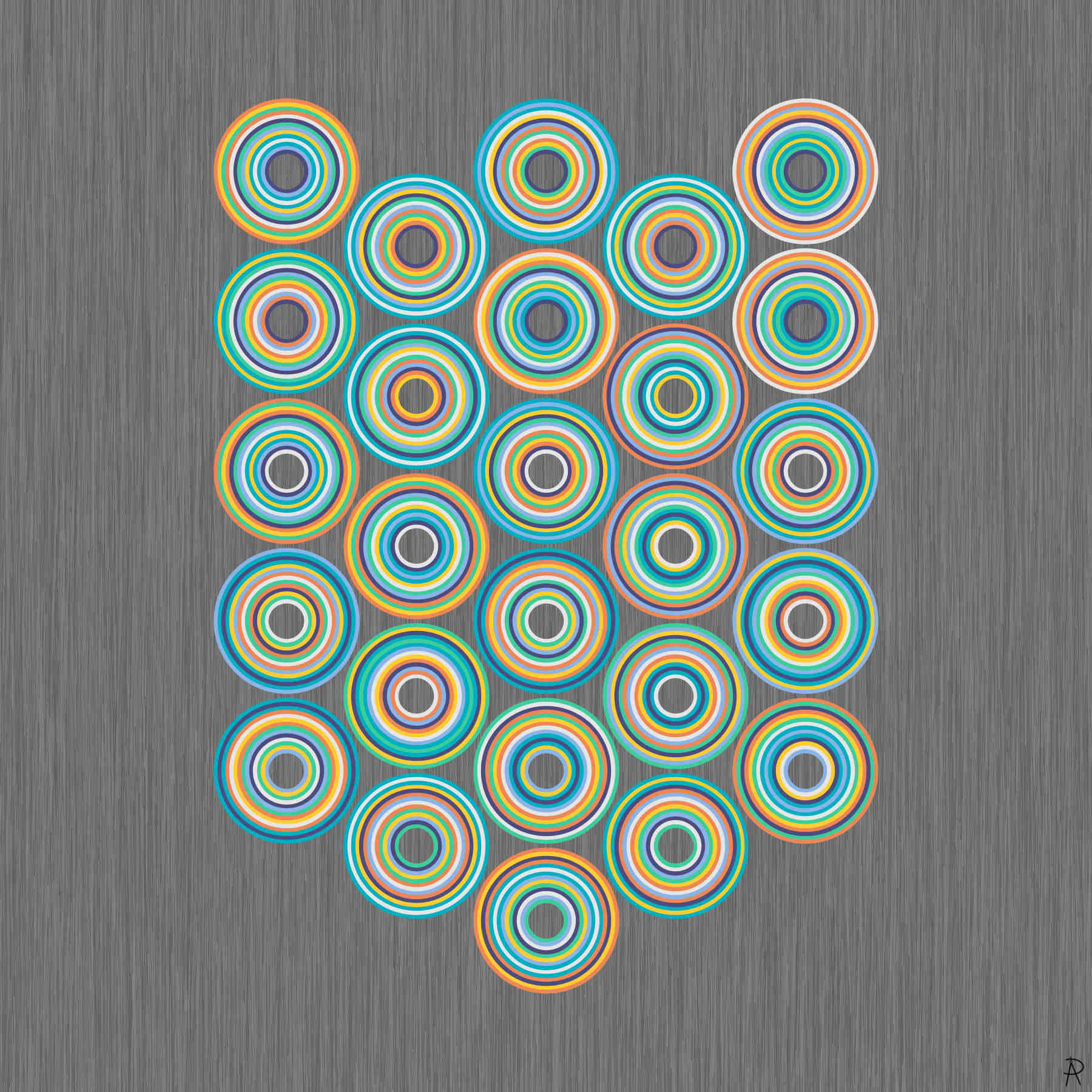Archipelago of Octadics Digital Print 2021

This artwork answers the question of how many ways can one place eight color pairs such that there is one other color between the first color pair, two colors between the second color pair, and so on. Mathematically, these are the twenty-six different Langford sequences for $$n = 8$$. This piece is the fourth in a series of artworks related to Langford sequences.

### Background and Inspiration

A Langford sequence, named for C. Dudley Langford (October 16, 1905 – January 11, 1969), is a permutation of the integers $$1, 1, 2, 2, 3, 3, \ldots, n, n$$ for some positive integer $$n$$ where the gap between the first and second occurrence of a value $$k$$ is $$k$$. These sequences are typically written as a juxtaposition of the digits for small values of $$n$$. There is one Langford sequence of order 3 (312132) and one of order four (41312432). A Langford sequence and its reverse are considered equivalent.

Langford's account of the origin of these sequences was published in 1958:

Years ago, my son, then a little boy, was playing with some coloured blocks. There were two of each colour, and one day I noticed that he had placed them in a single pile so that between the red pair there was one block, two between the blue pair, and three between the yellow. I then found that by a complete rearrangement I could add a green pair with four between them.

Langford went on to provide several examples of these sequences, with colors replaced with integers representing the number of colors between two identical colors. He invited others to provide a theoretical understanding of these sequences.

In 1959, Davies proved a Langford sequence exists when its length is $$4m$$ or $$4m-1$$ for positive integer $$m$$. Thus, there are no Langford sequences of lengths 1, 2, 5, 6, 9, 10, etc.

Martin Gardner's Mathematical Games column from the November 1967 Scientific American gave new attention on these sequences. Computer searches yielded new results on the number of sequences for a given $$n$$.

The number of sequences of a given length rises rapidly. There are 26 such sequences when $$n = 7$$, 150 sequences when $$n=8$$, and 17792 sequences when $$n=11$$.

Langford sequences are related to Skolem sequences. I became aware of these sequences at a Math/CS colloquium talk given by Heather Jordon at Albion College in 2012. Artistically, I was inspired by Bridget Riley's stripe paintings, such as Cool Edge (1982), through a talk by Neil Dodgson at the Bridges Enschede conference (2013).

### Related WorksLangford's Blocks Digital Print, 2021 This work depicts the origins of Langford sequences as described above and illustrates the unique Langford sequence of order 3.Chevronels Digital Print, 2021 This work depicts the origins of Langford sequences as described above and illustrates the unique Langford sequence of order 4.Septenary Circles Digital Print, 2021 This work depicts the Langford sequences of order 7.

### References

• C. Dudley Langford. Problem. The Mathematical Gazette, vol. 42, no. 341, 1958, pp. 228–228. JSTOR, www.jstor.org/stable/3610395.
• In November 1967, Martin Gardner, Mathematical Games: A mixed bag of logical and illogical problems to solve, Scientific American, November 1967.
• Roy O. Davies. On Langford's Problem (II). The Mathematical Gazette, vol. 43, no. 346, 1959, pp. 250–255. JSTOR, www.jstor.org/stable/3610650.
• Neil Dodgson, Mathematical characterization of Bridget Riley's stripe paintings, Journal of Mathematics and the Arts, Volume 6, Issue 2--3, 2012.
• Sequence A014552 in the On-Line Encyclopedia of Integer Sequences (OEIS.org).
• E.A.M., Obituary: C. Dudley Langford, The Mathematical Gazette, vol. 53, no. 385, December 1969, 314.